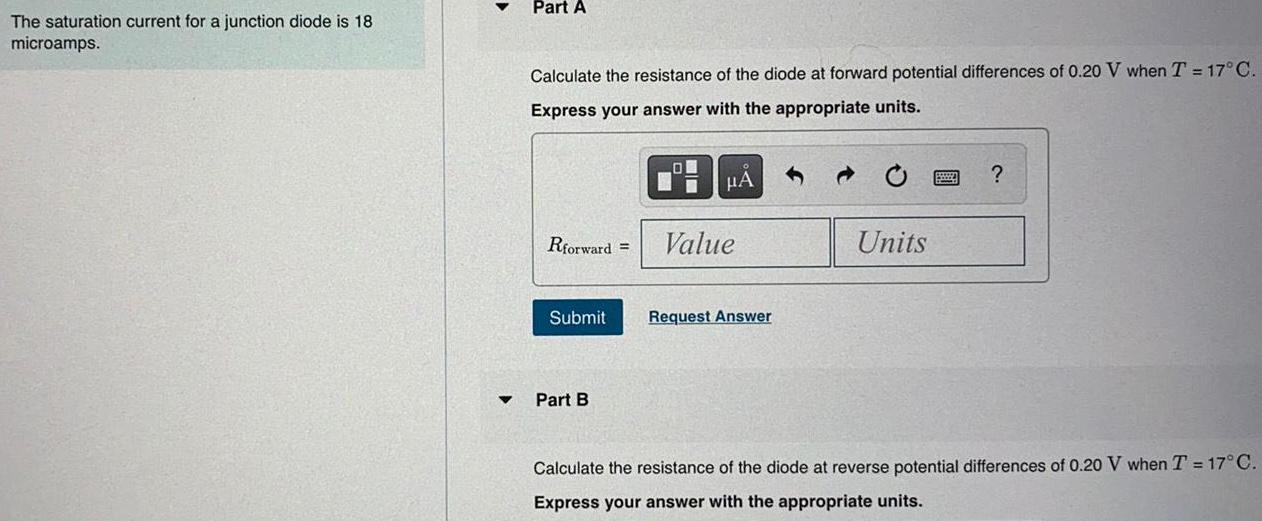Question:

# The saturation current for a junction diode is 18 microamps.

Last updated: 7/10/2022The saturation current for a junction diode is 18 microamps. Calculate the resistance of the diode at forward potential differences of 0.20 V when T = 17°C. Express your answer with the appropriate units. Calculate the resistance of the diode at reverse potential differences of 0.20 V when T = 17°C. Express your answer with the appropriate units.